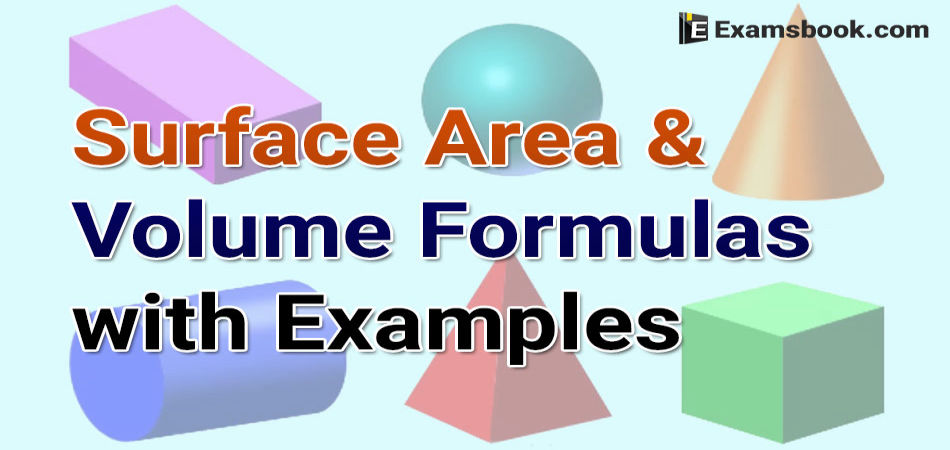• Save

# Surface Area and Volume Formulas with Examples for SSC and Bank Exams

Vikram Singh3 years ago 4.9K ViewsIn this blog, students who are preparing for ssc or bank exams can understand easily surface area and volume formulas. Students often face difficulty in these type of formulas in competitive exams. So, here I am providing important surface area and volume formulas which formulas asked mostly in competitive exams.

So you should practice surface area and volume questions with the help of these formulas and examples, after this try to solve yourself these questions with different equations. Click here for more practice of surface area and volume questions with answers.

## Surface Area and Volume Formulas for Competitive Exams

Surface Area

Surface area is the total area that can be measured on the entire surface. This can only be measured, if the object is a three-dimensional object. Surface area is measured in square unit.

Volume

Volume is the space occupied by an object considering three-dimensional view. The formulas for calculating volume vary with the shape of the object. The units of volume in metric are cubic meters, cubic centimetres etc.

Important formulae

I. Cuboid

Let length = l, breadth = b and height = h units. Then,

1. Volume = (l × b × h) cubic units.

2. Surface area = 2(lb + bh + lh) sq. units.

$$3.Diagonal =\sqrt {l^2+b^2+h^2 } \ units.$$

II. Cube

Let each edge of a cube be of length a. Then,

1. Volume = a3 cubic unit

2. Surface area = 6a2 sq. units.

3. $$Diagonal=\sqrt { 3}\ a \ unit$$

III. Cylinder

Let radius of base = r and Height (or length) = h. Then,

1. Volume = (πr2h) cubic units.

2. Curved surface area = (2πrh) sq. units.

3. Total surface area = (2πrh+2πr2) sq. units.

= 2πr (h +r) sq. units.

IV. Cone

Let radius of base = r and Height = h. Then,

$$1.Slant \ height,l =\sqrt {h^2+r^2 }\ units$$

$$2.Volume = \left({1\over3}\pi r^2h \right)cubics\ units$$

3. Curved surface area = (πrl) sq. units.

4. Total Surface area = (πrl+πr2) sq. units.

V. Sphere

Let the radius of the sphere be r. Then,

$$1.Volume = \left({4\over3}\pi r^3 \right)cubic\ units$$

2. Surface area = (4πr2) sq. units.

VI. Hemisphere

Let the radius of a hemisphere be r. Then,

$$1.Volume = \left({2\over3}\pi r^3 \right)cubic\ units$$

2. Curved surface area = (2πr2) cubic units.

3. Total surface area = (3πr2) sq. units.

Remember: 1 litre = 1000 cm3.

Solved examples

Ex.1. Find the volume and surface of a cuboid 16 m long, 14 m broad and 7 m high.

Solution

Volume = (16×14×7)m3 = 1568 m3

Surface area = [2(16×14+14×7+16×7)] cm2 = (2×434) cm2 = 868 cm2.

Ex.2. Find the length of the longest pole that can be placed in a room 12 m long, 8 m broad and 9 m high.

Solution

Length of longest pole = Length of the diagonal of the room

$$=\sqrt {12^2+8^2+9^2 } \ = \sqrt { 289} =17 \ m\$$

Ex.3. The volume of a wall, 5 times as high as it is broad and 8 times as long as it is high, is 12.8 cu. metres. Find the breadth of the wall.

Solution

Let the breadth of the wall be x metres.

Then, Height = 5x metres and Length = 40x metres.

$$∴ x×5x×40x=12.8⟺x^3={12.8\over200}={128\over2000}={64\over1000}$$

$$So, \ x={4\over10}\ m = \left({4\over10}×100 \right)cm = 40 \ cm$$

Ex.4. Find the number of bricks, each measuring 24 cm × 12 cm × 8 cm, required to construct a wall 24 m long, 8m high and 60 cm thick, if 10% of the wall is filled with mortar ?

Solution

Volume of the wall = ( 2400 × 800 × 60) cu. cm.

Volume of bricks = 90% of the volume of the wall

$$= \left({90\over100}×2400×800×60 \right) cu.cm.$$

Volume of 1 brick = (24×12×8) cu. cm.

$$∴ \ Number \ of \ bricks = \left({90\over100}×{2400×800×60\over24×12×8} \right)=45000$$

Ex.5. Water flows into a tank 200 m × 150 m through a rectangular pipe 1.5 m × 1.25 m @ 20 kmph. In what time (in minutes) will the water rise by 2 metres?

Solution

Volume required in the tank = (200×150×2) m3 = 60000 m3

Length of water column flown in 1 min

$$=\left({20×1000\over60} \right) m={1000\over3}m.$$

Volume flow per minute =

$$= \left(1.5×1.25×{1000\over3}\right)m^3=625 \ m^3$$

$$∴ Required \ time = \left({60000\over625} \right) min=96 \ min$$

I hope these formulas are helpful for you. If you have any problems or want to ask anything regarding surface area and volume formulas examples, you can ask me in the comment section.

All the best for your exam